×
Log in to StudySoup
Get Full Access to Purdue - ECE 202 - Class Notes - Week 1
Join StudySoup for FREE
Get Full Access to Purdue - ECE 202 - Class Notes - Week 1

Already have an account? Login here
×
Reset your password

# ece 202 purdue Description

##### Uploaded: 03/01/2017
17 Pages 189 Views 0 Unlocks
Reviews

LECTURE 1 : LAPLACE TRANSFORM BASICS—PART 1 1. Motivation and Solution Strategy (a) Question: What is Laplace Transform  Analysis? Answer 1: an algebraic technique for  computing responses of circuits/differential-equations with and without IC’s for a very large class of  practical input signals (voltage and current source  excitations). Comment: 201 provides responses only for constant  inputs for first and second order circuits along with  some sinusoidal steady state analysis. Now that will  get you a great job at NASA.(b) How is this “response calculation”  accomplished? Input Signal (voltage/current source) Circuit /Model h(t): Impulse Responseece 40100 textbook notes
If you want to learn more check out political order is the patterns or regularities of social behavior that are induced or set by authority relationships or coercion.
Don't forget about the age old question of math 207 iowa state
We also discuss several other topics like writing intensive mizzou
able>

## What do they tell you in calculus?## (b) How is this “response calculation” accomplished?## (a) Question: What is Laplace Transform Analysis?Don't forget about the age old question of srm university strength
Don't forget about the age old question of unlv math courses

→→Output Signal (voltage/current) ↓ ! ! L[Circuit /Model] H(s): Transfer Function

L[Input Signal] →→ L[Output Signal]2. Basic Signals ⎧⎨⎩ (a) Unit step function: u(t) = 1 t ≥ 0 0 t < 0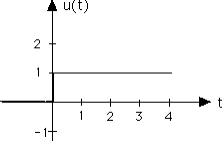Right Shifted Step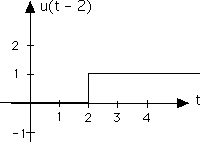Left Shifted StepFlipped Step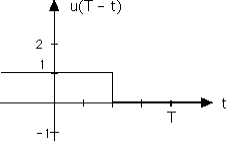t ∫ .  (b) Ramp function: r(t) = tu(t) = u(τ )dτ −∞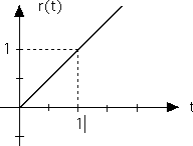Flipped and Shifted Ramp (Should be r(T − t) )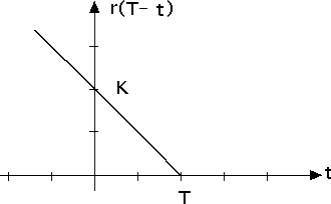(c) Delata function: δ (t) is defined as that  t ∫ .  “functional” satisfying u(t) = δ (τ )dτ −∞ INTERPRETATION:  ⎧⎨⎩ δ (t) = ddtu(t) = 0 t ≠ 0 ∞ t = 0 and 0+ ∫ = 1 AREA = δ (τ )dτ 0− ∞ ∫ = f (T ) SIFTING PROPERTY: f (τ )δ (τ − T )dτ −∞ Remark: the delta function has infinite height, zero  width, and a well defined area of 1 unit. ☺3. Decomposition of Graphical Signals into basic  steps, ramps, and shifts thereof. Example 1. Express f (t) as a sum of steps and  shifted steps.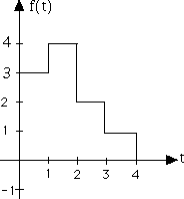f (t) = 3u(t)+ u(t −1)− 2u(t − 2)− u(t − 3)− u(t − 4)Example 2. Express f (t) as a sum of ramps and  shifted ramps.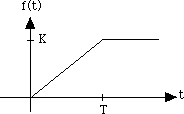f (t) = KTr(t)− KTr(t − T ) Example 3. Express f (t) as a sum of basic signals  and shifts thereof.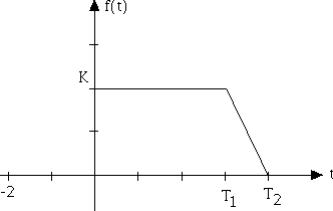f (t) = Ku(t)− K T2 − T1r(t − T1)+K T2 − T1r(t − T2 )4. Definition of the Laplace Transform of a Signal, a  Function, or an Excitation.  ∞ F(s) = L[ f (t)] ! f (t)e−st dt ∫ 0− where  (i) s = σ + jω is a complex variable;  (ii) the integral converts a function of time, f (t),  into a new function, F(s), dependent on a NEW  complex (NON-TIME) variable s. (iii) The complex variable s represents  “frequency”. (iv) Music signals are composed of notes of  different frequencies. Music signals over windows of  time then have many frequencies of “sines” and  “cosines” that determine the signal. A prism breaks  up white light into multi-colored light. The Laplace  transform is the prism and the variable s tells of the  many different colors.Example 4. Compute Delta(s) ∞ Delta(s) = L[δ (t)] ! δ (t)e−st dt ∫ = e−st ⎤⎦t=0 = 1 0− Example 5. Compute U(s) = L[u(t)] ∞ U(s) = L[u(t)] ! u(t)e−st dt ∞ ∫ = e−st ⎤ ∞ ∫ = e−st dt −s ⎦⎥0− ⎤ ⎦⎥0−= 1s NOTE: e−st ⎤ 0− = e−st −s 0− ⎦⎥t→∞− e−st ⎤ −s −s ⎦⎥t→∞= 0 ALWAYS in 202 for reasons  to be explained in lecture 2.Example 6. Compute R(s) = L[r(t)] ∞ ∞ ∞ R(s) = L[r(t)] ! tu(t)e−st dt ∫ = − ddse−st ( )dt ∫ = te−st dt ∫ 0− ∞ 0− ∞ ⎛ 0− ⎞ ∫ = − ddse−st ⎡ = − ddse−st dt 0− ⎜⎜ ⎝ ⎣⎢ ⎤⎦⎥0− −s ⎟⎟= − dds1s⎛⎝⎜ ⎞⎠⎟ = 1s2⎠ 5. Some Practical Theorems—for Simplifying  Calculations (a) Linearity Theorem:  L a1 f1(t)+ a2 f2 [ (t)] = a1L f1 [ (t)]+ a2L f2 [ (t)] = a1F1(s)+ a2F2 (s) Proof: Follows directly from the distributive property  of the integral.(b) Time Shift Theorem:  L[ f (t − T )u(t − T )] = e−sT F(s) Proof:  Step 1. Write down definition: F(s) = L[ f (t − T )u(t − T )] ∞ ∞ ! f (t − T )u(t − T )e−st dt ∫ = f (t − T )e−st dt ∫ 0− T − Step 2. What do they tell you in calculus? Change of  variable??? (a) τ = t − T ⇒ t = τ + T (b) τ = 0− when t = T − (c) dt = dτStep 3. Substitute …. ∞ ∞ L[ f (t − T )u(t − T )] = f (t − T )e−st dt ∫ = f (τ )e−s(τ +T )dτ ∫ ∞ T − 0− = e−sT f (τ )e−sτ dτ ∫ = e−sT F(s)0− 5. OTHER EXAMPLES Example 7. Compute F(s).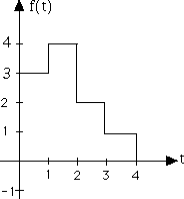f (t) = 3u(t)+ u(t −1)− 2u(t − 2)− u(t − 3)− u(t − 4) Using Linearity, the shift theorem, and the fact that  L[u(t)] = 1s: F(s) = 3s+e−ss − 2 e−2s s − e−3s s − e−4s sExample 8. Compute F(s).f (t) = KTr(t)− KTr(t − T ) Using Linearity, the shift theorem, and the fact that  L[r(t)] = 1s2 : F(s) = KT⋅1s2 − KT⋅e−Ts s2Example 9. Compute F(s).f (t) = Ku(t)− K T2 − T1r(t − T1)+K T2 − T1r(t − T2 ) By inspection using the linearity and time shift  theorems: T2 − T1⋅e−T1s T2 − T1⋅e−T2s F(s) = Ks − K s2 +K s2
Page ExpiredIt looks like your free minutes have expired! Lucky for you we have all the content you need, just sign up here